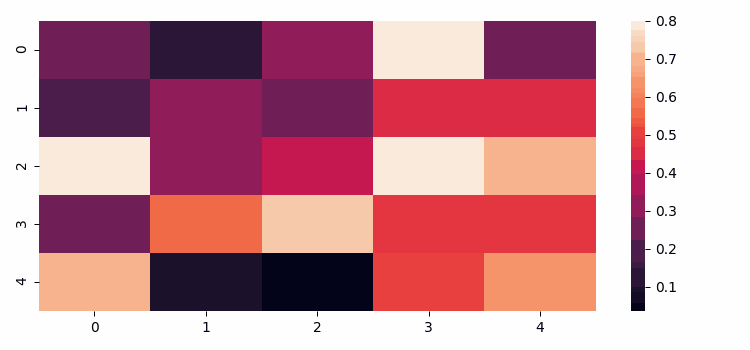# How to animate a Seaborn heatmap or correlation matrix(Matplotlib)?

To animate a Seaborn heatmap or correlation matrix, we can take the following steps −

• Set the figure size and adjust the padding between and around the subplots.
• Create a new figure or activate an existing figure.
• Make a dimension tuple.
• Make a Seaborn heatmap.
• Create an init() method for the first heatmap.
• Use FuncAnimation() class to make an animation by repeatedly calling a function animate that will create a random dataset and create a heatmap.
• To display the figure, use show() method.

## Example

import numpy as np
import seaborn as sns
import matplotlib.pyplot as plt
from matplotlib import animation

plt.rcParams["figure.figsize"] = [7.50, 3.50]
plt.rcParams["figure.autolayout"] = True

fig = plt.figure()
dimension = (5, 5)
data = np.random.rand(dimension, dimension)
sns.heatmap(data, vmax=.8)

def init():
sns.heatmap(np.zeros(dimension), vmax=.8, cbar=False)

def animate(i):
data = np.random.rand(dimension, dimension)
sns.heatmap(data, vmax=.8, cbar=False)

anim = animation.FuncAnimation(fig, animate, init_func=init, frames=20, repeat=False)

plt.show()

## Output